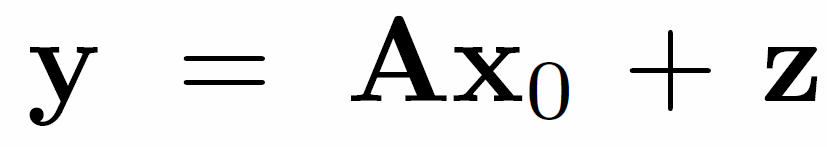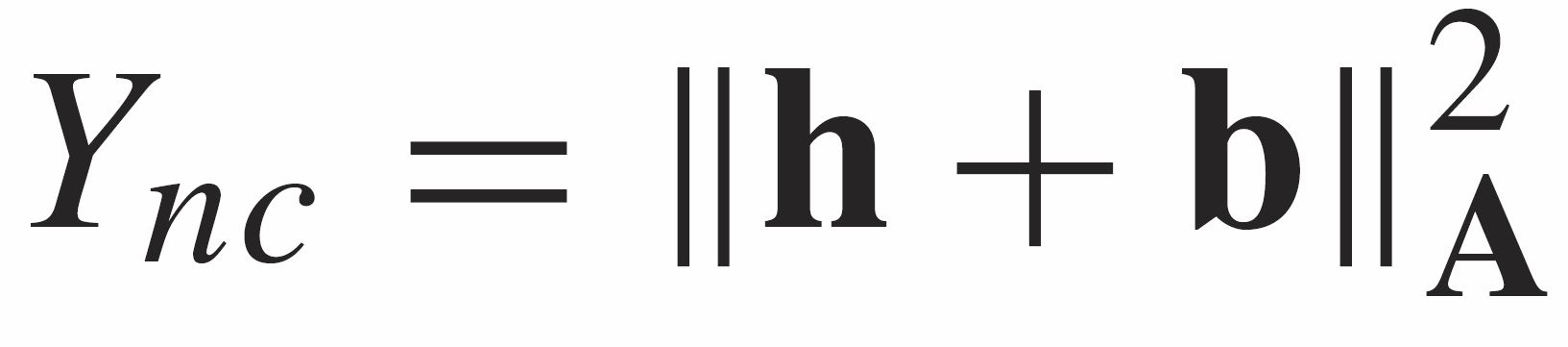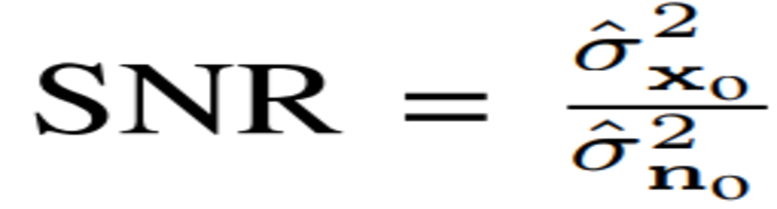•### Constrained Perturbation Regularization Approach for Signal Estimation Using Random Matrix Theory

This software is a new regularization approach and a new regularization parameter selection approach for linear least squares discrete ill-posed problems.

lease send Comments to: Mohammed Suliman

Software Describtion:

Estimating the values of unknown parameters from corrupted measured data faces a lot of challenges in ill-posed problems. In such problems, many fundamental estimation methods fail to provide a meaningful stabilized solution. This software is a new regularization approach and a new regularization parameter selection approach for linear least squares discrete ill-posed problems. It is based on enhancing the singular-value structure of the ill-posed model matrix to acquire a better solution.

Unlike many other regularization algorithms that seek to minimize the estimated data error, this algorithm is developed to minimize the mean-squared error of the estimator which is the objective in many typical estimation scenarios.

The performance of the proposed approach is demonstrated by applying it to a large set of real-world discrete ill-posed problems. Simulation results demonstrate that the proposed approach outperforms a set of benchmark regularization methods in most cases. In addition, the approach also enjoys the lowest runtime and offers the highest level of robustness amongst all the tested benchmark regularization methods.

References:

• Mohamed A. Suliman, Tarig Ballal, and T. Y. Al-Naffouri, "Perturbation-Based Regularization for Signal Estimation in Linear Discrete Ill-Posed Problems", in Signal Processing, Feb. 2018.

•### On the Distribution of Indefinite Quadratic Forms in Gaussian Random Variables

This software evaluate the CDF and PDF of indefinite quadratic forms in Gaussian random variables.

Please send commnets to Muhammed Moinuddin

Software Discribtion:

This software evaluate the CDF and PDF of indefinite quadratic forms in Gaussian random variables. Such a quantity appears in many applications in communications, signal processing, information theory, and adaptive filtering. For example, this quantity appears in the mean-square-error (MSE) analysis of the normalized least-mean square (NLMS) adaptive algorithm, and SINR associated with each beam in beam forming applications.

The trick of this software is to replace inequalities that appear in the CDF calculation with unit step functions and to use complex integral representation of the unit step function. Complex integration allows us then to evaluate the CDF in closed form for the zero mean case and as a single dimensional integral for the non-zero mean case. Utilizing the saddle point technique allows us to closely approximate such integrals in non zero mean case.

The software be used for the joint distribution of quadratic forms and ratios of such forms, and to characterize quadratic forms in isotropic distributed random variables.

The code also evaluates the outage probability in multiuser beamforming using our  pproach to provide an application of indefinite forms in communications.

Reference:

• T. Y. Al-Naffouri and M. Moinuddin and N. Ajeeb and B. Hassibi and A. L. Moustakas, "On the Distribution of Indefinite Quadratic Forms in Gaussian Random Variables", IEEE Transactions on Communications.   vol. 64 , pp. 153-165, Jan 2016

•### SNR Estimation in Linear Systems With Gaussian Matrices

This work proposes a highly accurate algorithm to estimate the signal-to-noise ratio (SNR) for a linear system from a single realization of the received signal.

Software Discription:

This work proposes a highly accurate algorithm to estimate the signal-to-noise ratio (SNR) for a linear system from a single realization of the received signal. We assume that the linear system has a Gaussian matrix with one sided left correlation while unknown entries of the signal and the noise are assumed to be independent and identically distributed with zero mean and can be drawn from any distribution. We use the ridge regression function of this linear model in company with tools and techniques adapted from random matrix theory to achieve, in closed form, accurate estimation of the SNR without prior statistical knowledge on the signal or the noise.

References:

• M. A. Suliman, A. M. Alrashdi, T. Ballal, T. Y. Al-Naffouri, "SNR Estimation in Linear Systems with Gaussian Matrices", IEEE Signal Processing Letters, v. 24, issue 12, pp. 1867 - 1871, Dec. 2017Algorithm Performance

•### Support agnostic Bayesian matching pursuit for block sparse signals

SABMP is a compressive sensing algorithm that takes advantage of the sparsity feature of of the signals and the statistic of the noise to enhance the estimation performance.

Software Description:

Some signal processing problems can be modeled as finding a sparse solution to an under-determined linear system of equations. There are a number of ways to find the sparse solution. We considered a Matching Pursuit approach that is Bayesian in nature and at the same time agnostic to the distribution of the unknowns. Our formulation involves projection of observations onto the orthogonal complement space of the regression matrix elements. The proposed greedy approach and efficient computation strategies resulted in an algorithm that is faster than many similar algorithms. The algorithm performs better than other algorithms and has been applied to a vast array of problems from communications and image processing.

References:

• Mudassir Masood and T. Y. Al-Naffouri, "Sparse Reconstruction Using Distribution Agnostic Bayesian Matching Pursuit," in IEEE Transactions on Signal Processing, vol. 61, no. 21, pp. 5298-5309, Nov.1, 2013.
• Mudassir Masood and T. Y. Al-Naffouri, "Support agnostic Bayesian recovery of jointly sparse signals," 2014 22nd European Signal Processing Conference (EUSIPCO), Lisbon, 2014, pp. 1741-1745.
• Mudassir Masood and T. Y. Al-Naffouri, "Support agnostic Bayesian matching pursuit for block sparse signals," 2013 IEEE International Conference on Acoustics, Speech and Signal Processing, Vancouver, BC, 2013, pp. 4643-4647.
• Mudassir Masood, L. H. Afify and T. Y. Al-Naffouri, "Efficient Coordinated Recovery of Sparse Channels in Massive MIMO," in IEEE Transactions on Signal Processing, vol. 63, no. 1, pp. 104-118, Jan.1, 2015.